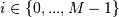# numpy.linalg.eig¶

numpy.linalg.eig(a)[source]

Compute the eigenvalues and right eigenvectors of a square array.

Parameters: a : (..., M, M) array Matrices for which the eigenvalues and right eigenvectors will be computed w : (..., M) array The eigenvalues, each repeated according to its multiplicity. The eigenvalues are not necessarily ordered. The resulting array will be always be of complex type. When a is real the resulting eigenvalues will be real (0 imaginary part) or occur in conjugate pairs v : (..., M, M) array The normalized (unit “length”) eigenvectors, such that the column v[:,i] is the eigenvector corresponding to the eigenvalue w[i]. LinAlgError If the eigenvalue computation does not converge.

eigvalsh
eigenvalues of a symmetric or Hermitian (conjugate symmetric) array.
eigvals
eigenvalues of a non-symmetric array.

Notes

Broadcasting rules apply, see the numpy.linalg documentation for details.

This is implemented using the _geev LAPACK routines which compute the eigenvalues and eigenvectors of general square arrays.

The number w is an eigenvalue of a if there exists a vector v such that dot(a,v) = w * v. Thus, the arrays a, w, and v satisfy the equations dot(a[:,:], v[:,i]) = w[i] * v[:,i] for.

The array v of eigenvectors may not be of maximum rank, that is, some of the columns may be linearly dependent, although round-off error may obscure that fact. If the eigenvalues are all different, then theoretically the eigenvectors are linearly independent. Likewise, the (complex-valued) matrix of eigenvectors v is unitary if the matrix a is normal, i.e., if dot(a, a.H) = dot(a.H, a), where a.H denotes the conjugate transpose of a.

Finally, it is emphasized that v consists of the right (as in right-hand side) eigenvectors of a. A vector y satisfying dot(y.T, a) = z * y.T for some number z is called a left eigenvector of a, and, in general, the left and right eigenvectors of a matrix are not necessarily the (perhaps conjugate) transposes of each other.

References

G. Strang, Linear Algebra and Its Applications, 2nd Ed., Orlando, FL, Academic Press, Inc., 1980, Various pp.

Examples

>>> from numpy import linalg as LA


(Almost) trivial example with real e-values and e-vectors.

>>> w, v = LA.eig(np.diag((1, 2, 3)))
>>> w; v
array([ 1.,  2.,  3.])
array([[ 1.,  0.,  0.],
[ 0.,  1.,  0.],
[ 0.,  0.,  1.]])


Real matrix possessing complex e-values and e-vectors; note that the e-values are complex conjugates of each other.

>>> w, v = LA.eig(np.array([[1, -1], [1, 1]]))
>>> w; v
array([ 1. + 1.j,  1. - 1.j])
array([[ 0.70710678+0.j        ,  0.70710678+0.j        ],
[ 0.00000000-0.70710678j,  0.00000000+0.70710678j]])


Complex-valued matrix with real e-values (but complex-valued e-vectors); note that a.conj().T = a, i.e., a is Hermitian.

>>> a = np.array([[1, 1j], [-1j, 1]])
>>> w, v = LA.eig(a)
>>> w; v
array([  2.00000000e+00+0.j,   5.98651912e-36+0.j]) # i.e., {2, 0}
array([[ 0.00000000+0.70710678j,  0.70710678+0.j        ],
[ 0.70710678+0.j        ,  0.00000000+0.70710678j]])


>>> a = np.array([[1 + 1e-9, 0], [0, 1 - 1e-9]])
>>> # Theor. e-values are 1 +/- 1e-9
>>> w, v = LA.eig(a)
>>> w; v
array([ 1.,  1.])
array([[ 1.,  0.],
[ 0.,  1.]])


numpy.linalg.svd

#### Next topic

numpy.linalg.eigh